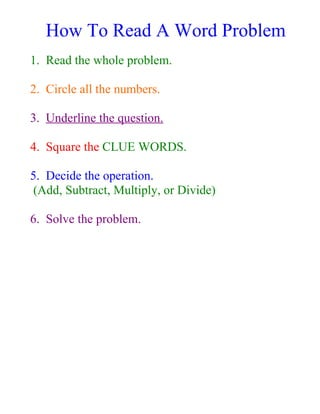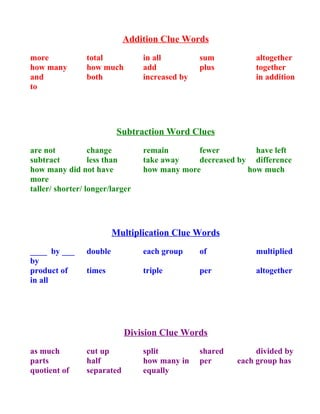Successfully reported this slideshow.

# How To Read A Word Problem 2 Pages

1

Share×
1 of 2
1 of 2

# How To Read A Word Problem 2 Pages

1

Share

## More Related Content

### Related Books

Free with a 14 day trial from Scribd

See all

### Related Audiobooks

Free with a 14 day trial from Scribd

See all

### How To Read A Word Problem 2 Pages

1. 1. How To Read A Word Problem 1. Read the whole problem. 2. Circle all the numbers. 3. Underline the question. 4. Square the CLUE WORDS. 5. Decide the operation. (Add, Subtract, Multiply, or Divide) 6. Solve the problem.
2. 2. Addition Clue Words more total in all sum altogether how many how much add plus together and both increased by in addition to Subtraction Word Clues are not change remain fewer have left subtract less than take away decreased by difference how many did not have how many more how much more taller/ shorter/ longer/larger Multiplication Clue Words ____ by ___ double each group of multiplied by product of times triple per altogether in all Division Clue Words as much cut up split shared divided by parts half how many in per each group has quotient of separated equally